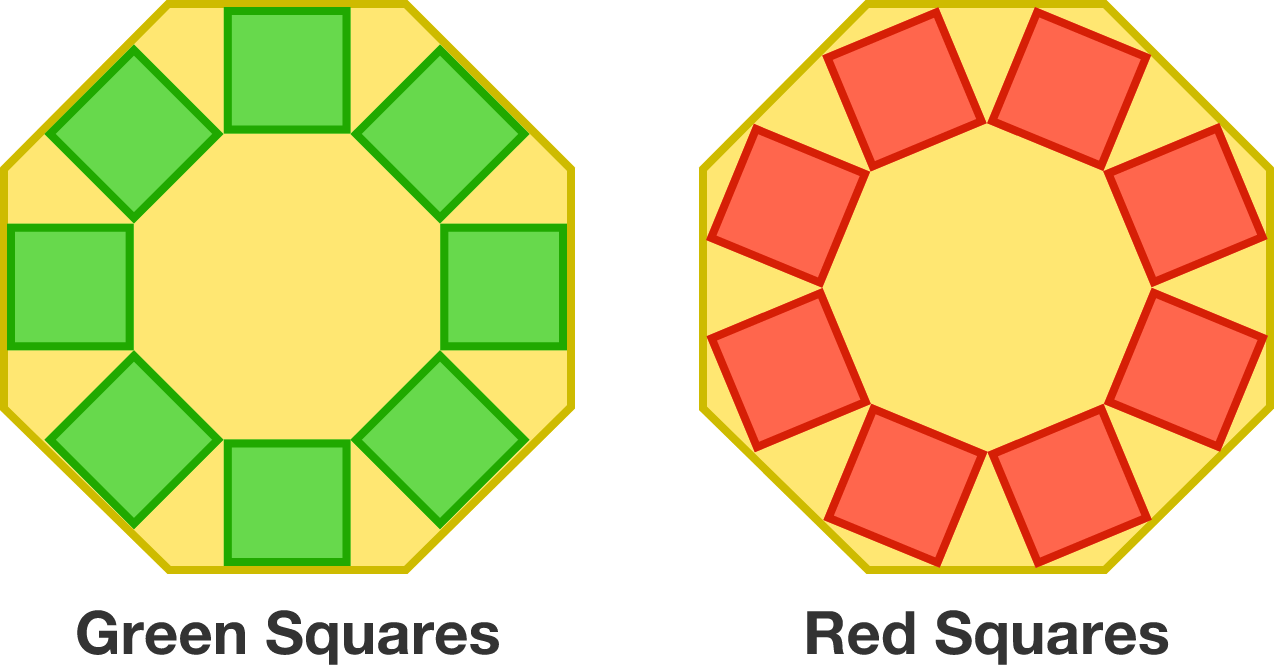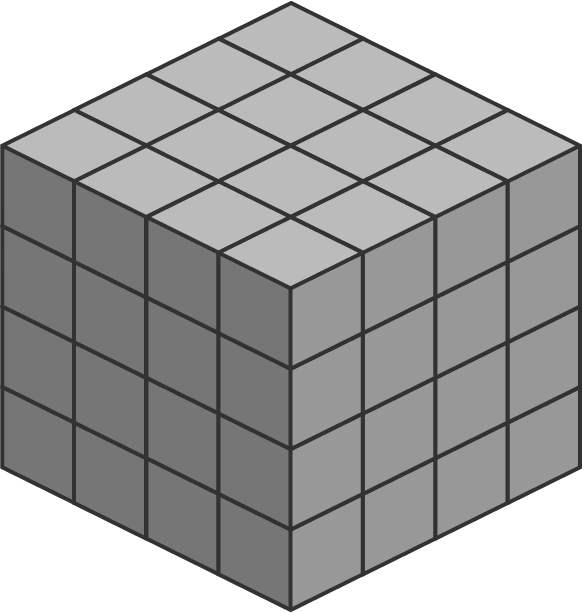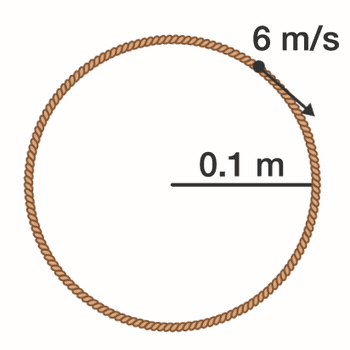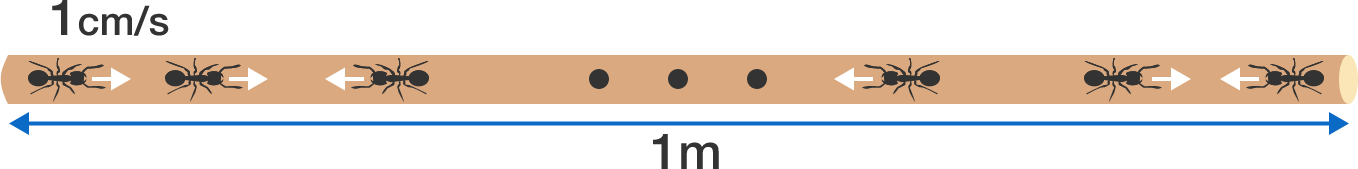# Problems of the Week

Contribute a problem

# 2017-08-14 IntermediateTwo sets of tangential, colored squares are positioned symmetrically in two identical regular octagons, as shown above.

• For the first octagon, each green square has one side lying on a side of the octagon.
• For the second octagon, each red square has two vertices touching two sides of the octagon.

Which set of squares is larger, green or red?

In a normal $3 \times 3$ tic-tac-toe board, there are 8 winning lines.How many winning lines are there in a $4\times 4\times 4$ tic-tac-toe board? (In this case, a winning line consists of four boxes in a row, either on the surface or inside the cube.)Bonus: How many winning lines are there in a $n^d$ tic-tac-toe hypercube, where $n$ is the number of cells per side and $d$ is the number of dimensions?

What is the sum of all positive integers less than $10^4$ which are relatively prime to $10^4?$A rope loop of radius $r = \SI[per-mode=symbol]{0.1}{\meter}$ and mass $m = 50\pi\,\text{g}$ rotates on a frictionless table such that the tangential velocity of any point on the loop is $v_\textrm{tangential}=\SI[per-mode=symbol]{6}{\meter\per\second}.$

Find the tension in the loop (in Newtons).

33 ants are placed on a pole of length $\SI{1.0}{\meter}.$ They each have negligible length, and they each crawl with a velocity of $\SI[per-mode=symbol]{1}{\centi\meter\per\second}.$ If two ants meet head on, they both turn around and immediately continue crawling.

If an ant reaches either end of the pole, the ant will drop off the pole. What is the longest possible time (in seconds) until all the ants drop off the pole?×